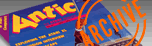`ANTIC VOL. 2, NO. 7 / OCTOBER 1983`

# ARITHMETRIX

## And now the amazing Atari!

by JERRY WHITE

Requires 16K RAM

Here is a simple ATARI BASIC program that demonstrates five arithmetic tricks. Arithmetrix can be used to fool your friends and make them think that your computer can read their minds.

Following is a sample run of the program, presented step by step. In each case, press [RETURN] after typing. Just read the screen, and answer the prompts as follows.

You will be asked to select a positive whole number. For all but the fourth case, use the number five for our first sample run.

(1) You are asked to multiply your number (minus one) by your number (plus one). We have chosen the number five so our number (minus one) equals four and our number (plus one) equals six. We multiply six by four and enter the result 24. The program tells us that our original number is five.

(2) You are asked to multiply your number by three, then tell the program if the result is odd or even. Our number is five, and five times three equals 15, so type ODD. We are asked to add one to the result (16), divide by two (eight), multiply by three (24), subtract six (18), then divide by nine and disregard any remainder (two). When you enter the result of two, the program will again tell you that your original number is five.

(3) You are asked to divide your original number by three, then enter the remainder. Five divided by three is one with a remainder of two, so type two. You are then asked to divide your original number by four and enter the remainder of one, then divide by five and enter the remainder of zero. Once again, the program has enough information to figure out your original number.

(4) This time the computer will predict your result. You are asked to select a three-digit number, and make sure that the difference between the first and last digits is greater than one. For this example, we will use the number 123. The first result is obtained by reversing the order of our number (321). The second result is obtained by finding the difference between our original number and the first result (198). Result number three is obtained by reversing the digits of the second result (891). You are asked to add the second result to result number three then press [RETURN]. Like magic, your computer will tell you that the final result is 1089.

(5) In the final algorithm the computer again predicts the answer. Choose a starting number, perhaps five. To obtain the first result we multiply by six (30), add 36 (66), divide by two (33). To obtain result number two we multiply our original number by three (15). Subtract result number two from the first result, press [RETURN], and your smart little machine will tell you that your final result is 18.

The program listing is divided into sections, each labeled with REM statements. It should be obvious that there isn't any magic at all. It's all BASIC logic.

The display routine reads from DATA statements and centers each new line on the screen. Since a comma indicates a new line of DATA, the "@" character was used in the data wherever a comma is to be displayed on the screen. The display routine then searches each line and converts each "@" to ",". My thanks to Sid Hyman for the idea for Arithmetrix.

Jerry White is Technical Consultant and regular contributor to ANTIC. His programs are available from several software companies, notably Adventure International and Educational Software.